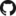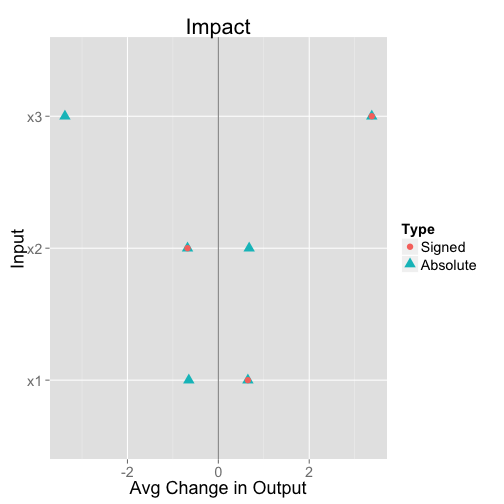# PredcompsHome

PDF Manual

Per Unit Input (original APC)

Impact (in units of output)

## Examples

Examples Overview

A fake logistic regression example predicting wine sales

A linear model with interactions

Loan defaults example

## More

As compared with Gelman & Pardoe 2007

Pairs & Weights

## Impact: A variation on APCs with comparable units

Impact answers the question: What difference does each input tend to make in the output? (Given the model and the distribution of inputs.) The units are the units of the output variable.

### The name “Impact”

First off, I don't love the name “Impact” for this. “Variable importance” may signal somewhat the right idea to people already familiar with other measures of variable importance, but this notion is (or can be) signed, whereas variable importance generally isn't.

### Impact

Impact is a statistic similar to the APC, but which addresses two issues I've had with APCs:

1. APCs are good for their purpose (determining the expected difference in outcome per unit change in input), but this doesn't tell me how important an input is to my predictions. The APC could be high while the variation in the input is so small that it doesn't make a difference.

2. Relatedly, APCs across inputs with different units have different units themselves and so are not directly comparable. The example in the paper (see p. 47) uses mostly binary inputs, so this is mostly not a problem there. But I'm not sure the other inputs in that example belong on the same chart, and I would like to visualize the influence .

Both (1) and (2) could be addressed by standardizing the coefficients before computing the APC, but this feels a bit ad hoc and arbitrary. Instead, I take the simpler and more elegant approach of just not dividing by the difference in inputs in the computation of the APC. That is, impact is just the expectation of

$$\Delta_f = f(u_2,v) - f(u_1,v)$$

under the same process as in the APC:

1. sample $$v$$ from the (marginal) distribution of the corresponding inputs
2. sample $$u_1$$ and $$u_2$$ independently from the distribution of $$u$$ conditional on $$v$$

The computed quantity is therefore the expected value of the predictive difference caused by a random transition for the input of interest. The units are the same as the output variable. This statistic depends on the model, the variation in the input of interest, and the relationship between that inputs and the other inputs.

### Example (cont.)

The same example used to demonstrate APCs demonstrates the difference between impact and APCs. Recall that the inputs ($$x_1$$, $$x_2$$, $$x_3$$) are independent, with

$y \sim 2x_1 - 2x_2 + x_3 + \mathcal{N}(0,.1)$

However, the variation in input $$x_3$$ is much larger than the others:

n <- 200
x1 <- runif(n = n, min = 0, max = 1)
x2 <- runif(n = n, min = 0, max = 1)
x3 <- runif(n = n, min = 0, max = 10)
y <- 2 * x1 + (-2) * x2 + 1 * x3
df <- data.frame(x1, x2, x3, y)
fittedLm <- lm(y ~ ., data = df)


We can then compute and plot the impact:

library(predcomps)
apcDF <- GetPredCompsDF(fittedLm, df = df)
PlotPredCompsDF(apcDF) + theme_gray(base_size = 18)The impact for $$x_3$$ is about 5 times the impact for $$x_1$$, which makes sense as $$x_3$$ varies on a scale that is 10 times as large but with a coefficient half as big.

apcDF

##    Input PerUnitInput.Signed PerUnitInput.Absolute Impact.Signed
## x1    x1                   2                     2        0.6500
## x2    x2                  -2                     2       -0.6783
## x3    x3                   1                     1        3.3765
##    Impact.Absolute
## x1          0.6500
## x2          0.6783
## x3          3.3765


The examples section goes through more interesting examples demonstrating more subtle features of how impact and APCs work.